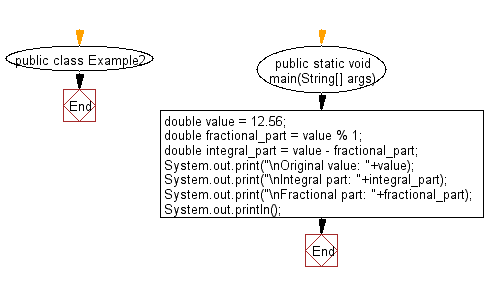﻿ Java Math Exercises: Get whole and fractional parts from a double value - w3resource# Java Math Exercises: Get whole and fractional parts from a double value

## Java Math Exercises: Exercise-2 with Solution

Write a Java program to get whole and fractional parts from a double value.

Sample Solution:

Java Code:

``````import java.util.*;
public class Example2 {
public static void main(String[] args) {
double value = 12.56;
double fractional_part = value % 1;
double integral_part = value - fractional_part;
System.out.print("\nOriginal value: "+value);
System.out.print("\nIntegral part: "+integral_part);
System.out.print("\nFractional part: "+fractional_part);
System.out.println();
}
}
``````

Sample Output:

```Original value: 12.56
Integral part: 12.0
Fractional part: 0.5600000000000005
```

Flowchart:Java Code Editor:

What is the difficulty level of this exercise?

﻿

## Java: Tips of the Day

Parsing dates:

```import java.io.*;
import java.util.*;
import java.text.*;

String s = "2001/09/23 14:39";

SimpleDateFormat formatter = new SimpleDateFormat ("yyyy/MM/dd H:mm");
Date d = formatter.parse(s, new ParsePosition(0));
```